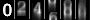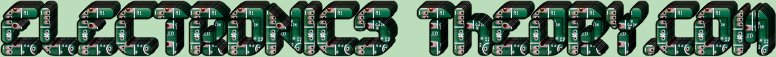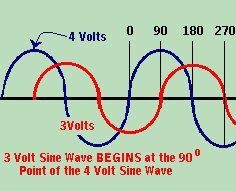ELI the ICE man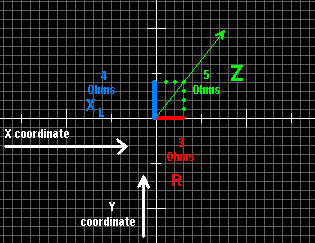In a previous lesson, we covered the fact that two alternating currents can be either in phase, or out of phase with respect to each other. We also discussed the addition of two sine waves of differing phase by using VECTOR ADDITION. I am fairly certain that you were hoping you would never see this again. Sorry, but you were SO wrong. We are soon going to get into the practical applications of vector addition. You are about to learn that in electronics, the capacitor and the inductor are exact opposites. The reason for this is because they BOTH store electricity, but in different ways. In a purely resistive circuit, there is no change in the phase from one component to another. When we add an inductor or capacitor into the circuit, however, the game changes completely, and the rules to the game are written with vectoral math.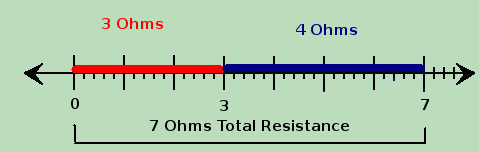Note that if we were to find the resistance of a series circuit with 2 resistors, one having 3 ohms, and the other having 4 ohms, we would simply add them, and come up with 7 ohms. If we were to graph this, we would have a single line along the "X" coordinate which is 7 units long, with points at 0, 3, and 7. If, however, we were to plot the Combined Resistance of a coil ( remember XL ? ) and a resistor we would have to plot a graph like the one above and to the left. This combined resistance is called IMPEDANCE, which is the TOTAL RESISTANCE TO THE FLOW of current. Note that Impedance is the TOTAL resistance to the flow, which includes "pure resistance" (from resistors), capacitive reactance, inductive reactance. The symbol for impedance is Z. If you have ever studied trigenometry, or even basic geometry, you may recall the formula for finding the hypotenuse of a right triangle ( A2+B2=C2). This will come in handy, as you compare it to the formula for impedance: R2+XL2=Z2 This can be re-written asNow let's assume that we have a series circuit like the one shown on the left.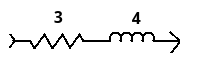Using the formula for IMPEDANCE ( Z ), R2 would be 32 which equals 9. XL2 would be 42 which would be 16. 9+16=25. The square root of 25 = 5, so the impedance of the circuit would be Z=5. Sometimes we might say that the "complex representation" of Z = R+Xj. In this case it would be 3+4j. This comes in handy as we begin adding capacitors into the circuit. Capacitors are like the opposite of inductors in a circuit. Whereas inductors are added ( Z = R + Xj ).... capacitors are subtracted (Z = R - Xj ). I know this all sounds confusing, but it will become clear as mud shortly. Recall the formula for Inductive Reactance? XL = 2πfL How could you forget? Well, CAPACITIVE REACTANCE is its opposite, and should also be memorized. Ready for this one?         1 XC = ----------------         2πfCWOW! It's almost the same formula! The only difference is that we substituted the L's for C's, and we reciprocated the formula (divided 1 by the formula). In the great scheme of things, that makes this formula not too difficult to remember, assuming you did memorize the formula for inductive reactance when I told you to. If you didn't, take time now to memorize both formulas. Your survival in electronics depends on them. Notice that I have flashed lots of formulas by you, but I have only asked you to memorize 3 of them... Ohm's Law, and the formula's for inductive and capacitive reactance. That is because you will use them over, and over again.Now let us examine our capacitive circuit. Once again, it has a resistance of 3, and a reactance of 4, but this time, it is a capacitive reactance, and not an inductive reactance. We will again use the formula for IMPEDANCE ( Z ), R2 would be 32 which equals 9. XC2 would be 42 which would still be 16. 9+16=25. The square root of 25 = 5, so the impedance of the circuit would once again be Z=5.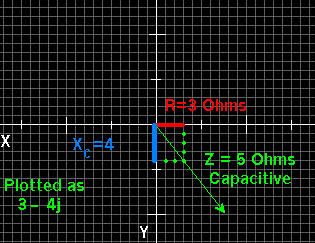But there is a catch - this time, because the circuit is CAPACITIVE, we would have a complex representation of impedance being equal to 3 - 4j. What exactly does this mean? It means that instead of plotting our graph in the POSITIVE direction along the Y axis of our graph, we would plot it in the NEGATIVE direction. Instead of our plotted point being (3,4) it would be located at (3,-4). I realize, of course, this is a lot of math to remember, but unless you are designing radio frequency, or other resonant circuits, you probably won't be using these formulas on a daily basis. You should be familiar with them though, and you SHOULD memorize the formulas I have pointed out thus far. One important point to keep in mind, is that when current flows through a purely resistive circuit, the voltage and current arrive at the same point at the same time. In other words, Voltage and Current are in phase in a purely resistive circuit. In a circuit which contains inductance or capacitance though this is not so. In an inductive circuit, the voltage leads the current by 90 degrees (assuming a purely inductive circuit). Likewise, in a capacitive circuit, the current leads the voltage by 90 degrees. Which leads which is easy to remember. Just think "Eli the Ice man". E=Voltage I=Current... L=Inductor......C=Capacitor ELI Inductive circuit...... Voltage arrives before Current . ICE Capacitive circuit... Current arrives before Voltage.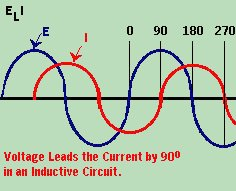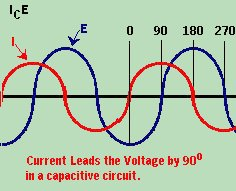(On The Following Indicator... PURPLE will indicate your current location) 1 2 3 4 5 6 7 8 9 10 11 12 13 14 15 16 17 18 19 20 21 22 23 24 25 26 27 28 29 30 31 32 33 34 35 36 37 38 39 40 41 42 43 44 45 46 47 48 49 50 51 52 53 54 55 56 57 58 59 60 61 62 63 64 65 66 67 68 69 70 71 72 73 74 75

[COURSE INDEX] [ELECTRONICS GLOSSARY] [HOME]Otherwise - please click to visit an advertiser so they know you saw their ad!

VISITORS: